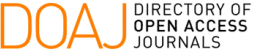### On High Order Barycentric Root-Finding Methods

Mario Meireles Graça, Pedro Miguel Lima

#### Abstract

To approximate a simple root of a real function f we construct a family of iterative maps, which we call Newton-barycentric functions, and analyse their convergence order. The performance of the resulting methods is illustrated by means of numerical examples.

#### Keywords

Order of convergence, Newton's method, Newton-barycentric map, nonlinear equations.

PDF

#### References

L. Collatz. Functional Analysis and Numerical Mathematics. Academic Press, New York (1966).

A. Cordero & J. Torregrosa. Variants of Newton’s method using fifth-order quadrature formulas. Appl. Math. Comput., 190 (2007), 686–698.

M. M. Graça & P. M. Lima. Root finding by high order iterative methods based on quadratures. Appl. Math. Comput., 264 (2015), 466–482.

M. M. Graça. Maps for global separation of roots. Electronic Transactions on Numerical Analysis, 45 (2016), 241–256.

Y. Ham, C. Chun & S.–G. Lee. Some higher-order modifications of Newton’s method for solving nonlinear equations. J. Comp. Appl. Math., 222 (2008), 477–486.

A. S. Householder. The Numerical Treatment of a Single Nonlinear Equation. McGraw-Hill, New York (1970).

H. T. Kung & J. F. Traub. Optimal order of one-point and multipoint iteration. J. Assot. Comput. Math., 21 (1974), 634–651.

G. Labelle. On extensions of the Newton-Raphson iterative scheme to arbitrary orders. Disc. Math Th. Comput Sc. (DMTCS), proc. AN, 845–856, Nancy, France (2010).

W. C. Rheinboldt. Methods for Solving Systems of Nonlinear Equations. 2nd Ed., SIAM, Philadelphia (1998).

P. Sebah & X. Gourdon. Newton’s method and high order iterations, 2001. Available from: http://numbers.computation.free.fr/Constants/constants.html.

G. Fernandez-Torres. Derivative free iterative methods with memory of arbitrary high convergence order. Numer. Alg., 67 (2014), 565–580.

J. F. Traub. Iterative Methods for the Solution of Equations. Prentice-Hall, Englewood Cliffs (1964).

S. Weerakoon & G. I. Fernando. A variant of Newton’s method with accelerated third-order convergence. App. Math. Lett., 13 (2000), 87–93.

S. Wolfram. The Mathematica Book. Wolfram Media, fifth ed., (2003).

DOI: https://doi.org/10.5540/tema.2016.017.03.0321

### Refbacks

• There are currently no refbacks.

Trends in Computational and Applied Mathematics

A publication of the Brazilian Society of Applied and Computational Mathematics (SBMAC)

Indexed in: